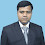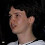## Saturday, May 28, 2011

### How to find if a number is perfect square

Number problems are abound in the programming interview world. A perfect square (also called a square number) is an integer that is the square of an integer; in other words, it is the product of some integer with itself. So, for example, 9 is a square number, since it can be written as 3 × 3. So are 16, 25, 49 and so on.

So how do we find if a given number is a square number or not? There are a many ways. This is a big research topic in the mathematics world. For the purpose of interview, we will not delve into highly complex solutions.

A bad implementation would be something like this:

`public static bool IsSquare(long target){    // loop through all the numbers till the target    for (long i = 0; i < target; i++)    {        // if we have a match        if ((i * i) == target)        {            return true;        }    }    // no matching number could be squared    return false;}`

This is an inefficient solution. There is no need to loop till the element. The only case when this would be true is 1. Beyond that, if a number is a square, it’s square root has to be less than half of the number (4 – 2, 9 – 3, 16 – 4, 25 – 5 an so on. Let’s modify our algorithm to add this logic.

`public static bool IsSquare(long target){    // loop through HALF the numbers till the target    for (long i = 0; i < target/2; i++)    {        // if we have a match        if ((i * i) == target)        {            return true;        }    }    // no matching number could be squared    return false;}`

Even though we have cut our loop by half, this is still inefficient. So what can we do more to stop the loop earlier. Let’s assume that our target number was 20. So using the code above, we will be looping till 10. Now once the loop crosses 5, we know that none of the numbers above that will result in the loop being true. So, a good condition to add would be break if the square of the current number is greater than the target. Let’s review this code below:

`public static bool IsSquare(long target){    // handle 0 and 1    if (target <= 1)        return true;    long currentSquare = 4;    long currentNumber = 2;    // loop through till the target is more than    // the square of the current number    while (currentSquare <= target)    {        // if we have a match, return true        if (currentSquare == target)            return true;        // increment current number        currentNumber++;        // find the next square        currentSquare = currentNumber * currentNumber;    }    // no matching number could be squared    return false;}`

Yes, this is still not the best solution but these code snippets should give you a good enough idea on how to optimize this algorithm further. I challenge the readers to take a few minutes out and come up with a better solution.

1.Im a kid. How would you explain it to me?

1.boova saappudu chellam.....:)

2.Add a check if the number does not end with 0,1,4,5,9 return false;

1.then 16 isnt a square. Idiot. :|

2.I made some tests here and noticed that using this method I loose some time in performance. Of course, this is a very tiny difference, but there are. However, I'm not sure if I built this if statement correctly... Seems like I have to do a lot of things to accomplish it.

else if (!Integer.toString(number).endsWith("1") || !Integer.toString(number).endsWith("4") ||
!Integer.toString(number).endsWith("5") || !Integer.toString(number).endsWith("6") ||
!Integer.toString(number).endsWith("9")){
return false;
}

3.You guys are trying way to hard.

public static bool IsSquare(long target)
{
return Math.Sqrt((double)target) % 1d == 0d;
}

1.seems good :)

2.yes it is good.But without using built in functions..
we need top go in other way that i Need .Thanks in advance

3.@Jacob
The point is in programming interviews you'll be asked not to used libraries. Otherwise, if the interviewer asks you to write a function for binary search, you could write:
return System.Array.BinarySearch(...);
Hahaha!

4.poda daiiiiiiii

5.This comment has been removed by the author.

6.This comment has been removed by the author.

7.Dear Nikhil Singhal,

Is he following a better solution in terms of efficiency??
Thank you.

int x;
bool flag=false;
cin>>x;
for (int i=2;i < x/2;i++)
{
if(x%i==0)
{
if (i*i==x)
{
flag=true;
break;
}
}

}

if(flag) cout<<"Perfect square";
else cout<>x;

8.You could do a binary search instead. Start with i = median(n). Then compare i^2 with n to determine which direction to go. Repeat until you have an i value such that i^2 == n or until you've exhausted all viable possibilities. Run-time is O(lg n) assuming multiplication is a constant-time operation.

1.Thanks. That really helped. Using a Binary Search never occured to me :)

9.private static bool IsSquare(long value)
{
double temp = Math.Sqrt(value);
return unchecked(temp == (int)temp);
}

1.sir, May i know how exact compare does between temp and int(temp), what double temp variable will stores.?

10.what about (x & (x-1)) == 0 ?

1.this is for checking if no. is a power of 2 or not

11.bool isPerfectSquare(long input)
{
// return true for trivial cases
if( input == 0 || input == 1)
{
return true;
}
else if( input == -1)
{
return false;
}

// make an initial guess and store as a floating point.
// the divisor is arbitrary, testing would have to be done to choose an optimal value
// basically the closer the initial value the faster the solution
double guess = input /2.0;

// uses approximation equation for 20 iterations or until the guess is close enough
// the max number of iterations could be adjusted
for(int i = 0; i < 20; i++)
{
// if the guess, is correct it will exit the loop.
if( guess * guess == input)
{
return true;
}

guess = 1.0/2.0 *( guess + input/guess);
}
return false;
}

12.public static boolean isSquare(int n)
{
int k=1;
while (n!=0)
{
if (n<0)
return false;

else
{
n=n-k;
k+=2;
}
}
return true;
}

13.This could be solved in a constant time O(C), given that integers have fixed bit-size. Digit-by-digit algorithm will have O(M) complexity, where M is number of bits in integer. Still the implementation is somewhat complicated.

14.public static bool isPerfectSquare(long target)
{
long result,perfSquare = target / 2;
do{
result = perfSquare * perfSquare;
if (result == target)
return true;
else if (target < result)
perfSquare = perfSquare - 1;
else
perfSquare = perfSquare / 2;
}while(target<result);
return false;
}

1.your looping as normal from half
Example : for 25 , your looping from 12,11,10.....5 (7 times)
programming should change to

public static bool isPerfectSquare(long target)
{
long result,perfSquare = target / 2;
do{
result = perfSquare * perfSquare;
if (result == target)
return true;
else if (result > target)
perfSquare = perfSquare / 2;
else
{
perfSquare = perfSquare * 2 - 1;
result = perfSquare * perfSquare;
if (result == target)
return true;
}
}while(target<result);
return false;
}

This allows to loop around values for 25 (12,6,3 then 5 result) 3 times..

15.One can keep adding odd integers starting from 1, until the sum matches the number (ansewer=yes) or exceedes (answer=no).
1 = 1^2
1+3 = 2^2
1+3+5 = 3^2
....
This is still a linear solution but addition is cheaper than multiplication.

16.public static bool IsSquare(int target)
{
for (int i = 0; i <= Math.Sqrt(target); i++)
{
System.Console.WriteLine(Math.Sqrt(target));
if (i * i == target)
{
return true;

}

}
return false;

}

better?

17.Here is how I did it using Binary Search.
Call the initial Binary Search with start =1;end = OriginalNumber/2 ; and original = OriginalNumber
bool binarySearch(long start,long end,long original)
{
long mid = (start + end) / 2;
if (original == 1)
return true;
if (mid * mid == original) { return true; }
if (start >= end)
return false;
if (mid * mid < original)
return binarySearch(mid + 1, end, original);
else
return binarySearch(start, mid - 1, original);

}

1.I am glad it helped you

18.Use just if(i*i<=target) and in inner if use as it is

19.Use just if(i*i<=target) and in inner if use as it is

20.function isSquare(n) {
return Math.sqrt(n) % 1 === 0;
}

This is easiest I found.

21.Wow! Such an amazing and helpful post this is. I really really love it. I hope that you continue to do your work like this in the future also.

Apache Spark Training in Pune
Spark Training Institute in Pune

22.23.24.You fully match our expectation and the selection of our data.
app designing company

25.Yes, You done really good work in this article. I love it. Everyone here and love your good work. Thanks for sharing this one. Now it's time to avail jupiter florida airport for more information.